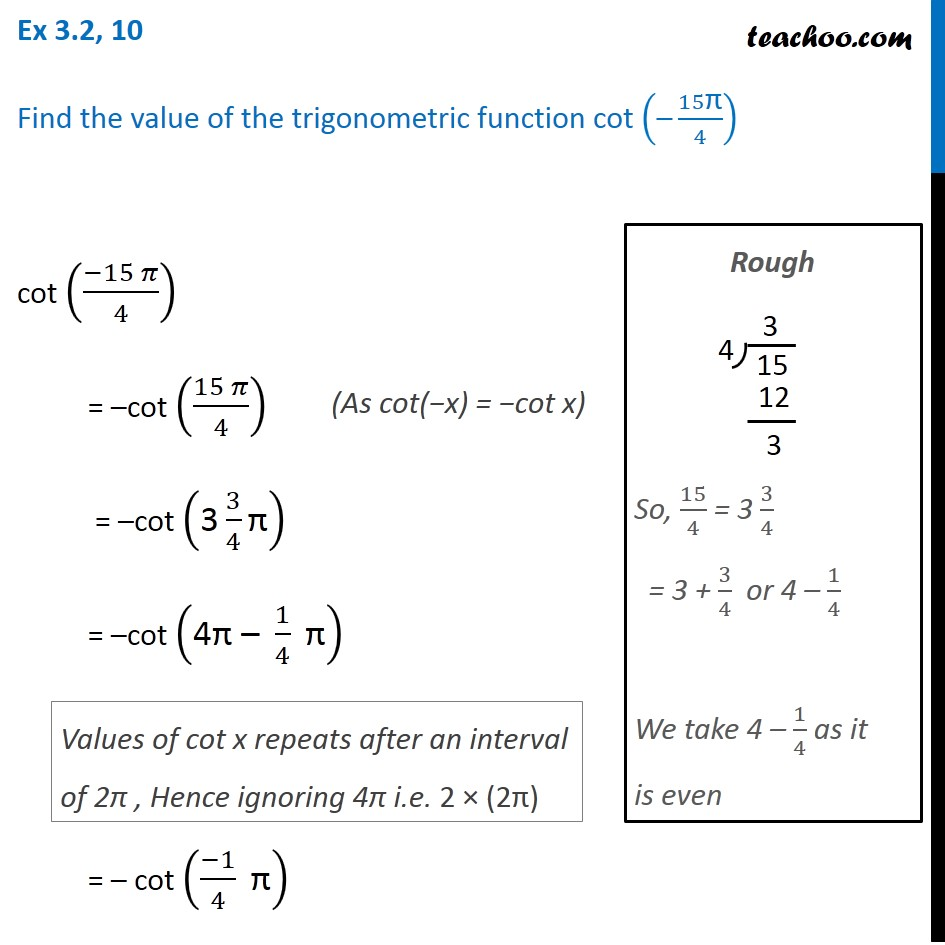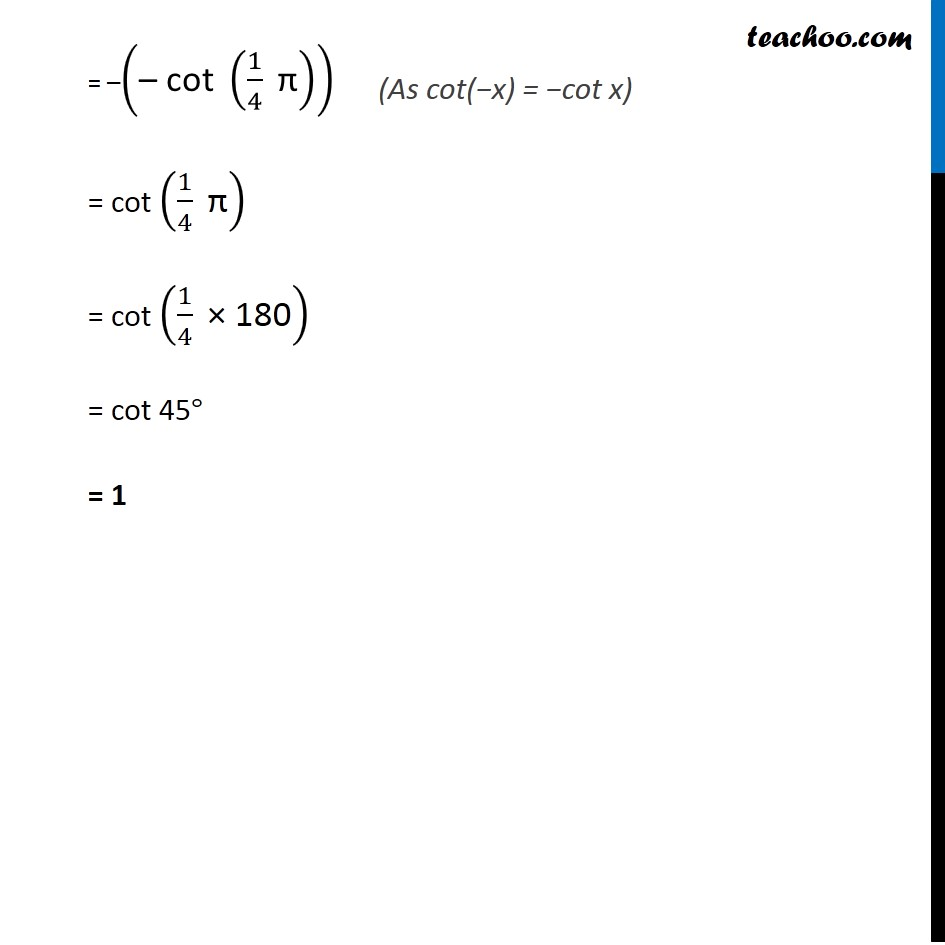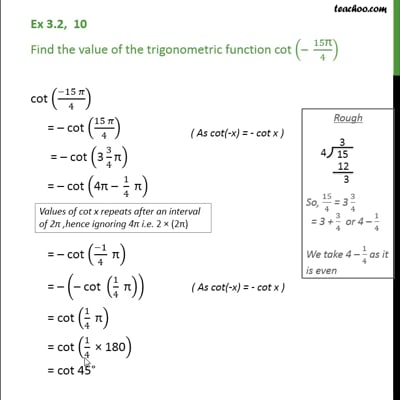Ex 3.2

Chapter 3 Class 11 Trigonometric Functions
Serial order wiseThis video is only available for Teachoo black users

Maths Crash Course - Live lectures + all videos + Real time Doubt solving!

### Transcript

Ex 3.2, 10 Find the value of the trigonometric function cot ("–" 15"π" /4) cot ((−15 𝜋)/4) = –cot ((15 𝜋)/4) = –cot ("3" 3/4 "π" ) = –cot ("4π – " 1/4 " π" ) = – cot ((−1)/4 " π" ) Values of cot x repeats after an interval of 2π , Hence ignoring 4π i.e. 2 × (2π) Rough So, 15/4 = 3 3/4 = 3 + 3/4 or 4 – 1/4 We take 4 – 1/4 as it is even = –("– cot " (1/4 " π" )) = cot (1/4 " π" ) = cot (1/4 " × 180" ) = cot 45° = 1